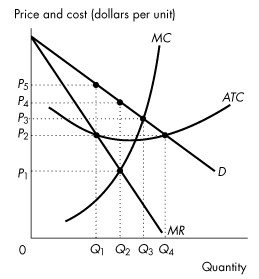/
/
/
21) Mrs. Smith's bakery shop a firm in monopolistic competition.
Not my Question
Flag Content

# Question : 21) Mrs. Smith's bakery shop a firm in monopolistic competition. : 1418019

21) Mrs. Smith's bakery shop is a firm in monopolistic competition. If in the short-run Mrs. Smith incurs an economic loss, she will keep producing as long as

A) price is less than average variable cost.

B) price is equal to marginal cost.

C) price is greater than or equal to average variable cost.

D) marginal revenue is greater than or equal to average variable cost.

E) marginal revenue is less than average variable cost.

22) Mrs. Smith's bakery shop is a firm in monopolistic competition. The firm's marginal revenue curve

A) is upward sloping.

B) is downward sloping and above the demand curve.

C) is a horizontal line.

D) is the same as the demand curve.

E) is downward sloping and lies below the demand curve.

23) Mrs. Smith's bakery shop is a firm in monopolistic competition. She is currently selling a box of bread for \$16. The firm's marginal cost is \$7 and marginal revenue is \$7. To maximize economic profit Mrs. Smith

A) shuts down.

B) decreases output so that marginal revenue exceeds marginal cost.

C) continues to produce the same level of output.

D) increases output so that marginal cost exceeds marginal revenue.

E) decreases output so that marginal cost equals the output price.

24) What will happen in the long run if firms in monopolistic competition incur economic loss?

A) Firms will continue producing and endure the losses.

B) Firms will leave the industry until the remaining firms make positive economic profit.

C) Firms will ask the government for financial aid.

D) Firms will leave the industry until the remaining firms make zero economic profit.

E) The level of investment in this industry will increase to boost the economy.

25) A firm has excess capacity if

A) it produces above its efficient scale.

B) it produces below its efficient scale.

C) it produces the same level as its efficient scale.

D) it sells some of its factors of production.

E) it improves the quality of its factors of production.

26) The real-estate industry is monopolistically competitive, so in this industry

A) the market demand curve is a horizontal line.

B) the market demand curve is upward sloping.

C) there are few realtors in the market.

D) excess capacity exists.

E) excess capacity does not exist.

27) A firm's markup is

A) the firm's total profit.

B) the firm's total cost.

C) the amount by which price exceeds marginal cost.

D) the amount by which price equals marginal cost.

E) the amount by which price is less than marginal cost.

28) Markup

A) does not exist in monopoly.

B) does not exist in monopolistic competition.

C) exists in perfect competition.

D) is the difference between price and average total cost.

E) exists in both monopoly and monopolistic competition.

29) In monopolistically competitive industries,

A) firms compete on quality, price and marketing.

B) the range of choice of products is the same as in perfectly competitive industries.

C) firms are insensitive to changes in consumer demand.

D) all firms produce a quantity at which marginal cost is greater than marginal benefit.

E) all firms make an economic profit.

30) Excess capacity in monopolistically competitive firms occurs because

A) each firm faces a demand that is perfectly elastic.

B) each firm builds a huge plant.

C) the existence of slightly differentiated products, serving almost the same purpose, causes a waste of precious natural resources.

D) firms produce an output that is less than the output at minimum average total cost.

E) marginal cost is too high.

Use the figure below to answer the following questions.Figure 14.2.4

## Solution 5 (1 Ratings )

Solved
Economics 1 Year Ago 25 Views
This Question has Been Answered!